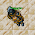## Wednesday, June 2, 2021

### TibiaGoals Riddles E9R02

Comment down below!

1.Respuesta: 2x3: 4 se convierte el 3 en 2 ... 2 x 2 : 4 ........... zazy torturador sombrio

2.Changing 3 into 2. 2x2=4
Eternal Hunger
Antica

3.Changing 3 into 2. 2x2=4
Eternal Hunger
Antica

4.Changing 3 into 2. 2x2=4
Eternal Hunger
Antica

5.2x2 = 4
Skyelie Hasulhoff <3

6.2X2= 4

Skyelie Hasulhoff <3

7.respuesta: 2x2=4
mover la flecha del 3 para convertirlo en 2
Chiki exotic

8.Respuesta 2x2 = 4 mover la flecha del 3 para convertir un 2
Wazonnick Exotic

9.10.2x2=4
Ray Jek

11.Move the bottom right line from the 3 to the west making it 2 times 2 = 4

Toxic Hasulhoff

12.Move the bottom right line from the 3 to the west making it 2 times 2 = 4

Toxic Hasulhoff

13.2x2=4 :D

Pinguzz

14.Anger Curse says:
Move the bottom right arrow of 3 to the left and make a 2
so 2x2=4

15.Hey! Move the lower right arrow of the 3 to the lower left of it so the equation says "2 x 2 = 4" :)

16.Change from 2x3=4 to 2x2=4 by moving arrow from 3 - the one pointing north(the lower one) from east to west
character name: Demonovsky

17.You move one arrow from the 3 to make it a 2, 2x2=4.

18.Nehkz
Relania

2x2=4 (moving the arrow from the 3 to generate a 2)

19.Nehkz
Relania

2x2=4 (moving the arrow from the 3 to generate a 2)

20.Move the bottom right arrow in the 3 to the left side making the equation 2x2=4

Xarkost Eilia

21.2 x 2 = 4

Shizurie

22.Moviendo la flechita del 3, para hacer la ecuación 2*2=4
By .Aurora Ventresca

23.Neo

By moving the bottom right vertical arrow from right to left, making the 3 a 2, so it would be 2 x 2 = 4

24.2x2 is 4 you can take the arrow from the 3 and move it bottom left, making the 3 into a 2 and thus correcting the equation

25.26.27.2*2=4... Bebitha consentida

28.2*2=4.... Bebitha consentida

29.2x2=4
Bloody Evan

30.2x2 = 4; Lord Neganek

31.2x2=4
Mian Stone'arrow

32.move lower east arrow pointing north from 3 to the west to change the equation from 2x3=4 to 2x2=4
name: Demonovsky

33.34.2x2=4
Lovely olka

35.By moving the bottom right arrow of the number 3 to the left so that the 3 forms a 2 instead. 2 x 2 = 4

-Essiee

36.The equation is: 2x2=4

Char name: Fyori

37.Turn the 3 into 2
2 x 2 = 4

Char: Dyaniixz

38.2 x 2 = 4

PAMELIIN

39.2x2 = 4! moving an arrow from 3 to make a 2!
character: pablo reformed

40.2 x 2 = 4
Character: Amy Meow

41.2x2=4/Norelli

42.2 x 2 = 4
samuray de carro :)

43.2 x 2 = 4
samuray de carro :)

44.Zuali:
https://i.gyazo.com/090485980d22e3249cc9e055b20ef846.png

45.